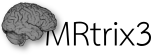Developer documentation Version 3.0.3-105-gd3941f44
MR::Math::ICLS Namespace Reference

functionality for solving constrained least-squares problems More...

class  Problem

class  Solver

## Detailed Description

functionality for solving constrained least-squares problems

the problem is to solve norm(Hx - b) subject to Ax >= t and optionally Bx = s. This sets up a class to be re-used and shared across threads, assuming the matrices H, A & B and vectors t & s don't change, but the vector b does.

The arguments passed to the Problem constructor correspond to:

• H - problem_matrix
• A - inequality_constraint_matrix
• B - equality_constraint_matrix
• b - problem_vector
• t - inequality_constraint_vector
• s - equality_constraint_vector

It is also possible to apply an additional minimum norm constraint that will be added to the problem cost function, to stablise ill-posed problems, and/or a separate minimum norm constraint on the Lagrangian multipliers to handle cases where they become degenerate (otherwise leads to errors in the Cholesky decomposition). Both default to zero, set one or both of them to a small value such as 1e-10 to help with these kinds of problem.

It is also possible to pass the problem already in standard form, whereby the matrix provided as problem_matrix is assumed to contain HTH (only its lower triangular part is taken into account), while the vectors provided to the solver will be assumed to contain HTb.